# Condition for Symmetry in Two Port Network

## Condition for Symmetry in Two Port Network:

If the impedance measured at one port is equal to the impedance measured at the other port with remaining port open circuited, the network is said to be Condition for Symmetry.

The impedances at two ports can be measured as shown in Fig. 6.6 (a) and (b).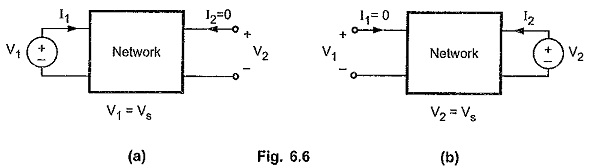#### Condition of Symmetry for z-Parameters:

The basic equations of z-parameters are as follows.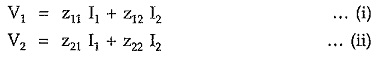Consider Fig. 6.6 (a).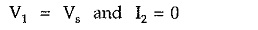Hence equation (i) will become,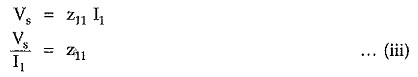Consider Fig. 6.6 (b).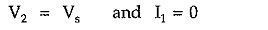Equation (ii) can be written as,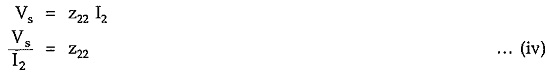For symmetrical networks, both the port impedances must be equal i.e.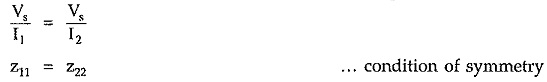#### Condition of Symmetry for y-Parameters:

The basic equations of y-parameters are as follows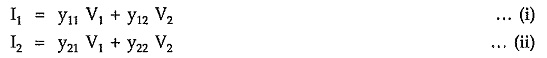Consider Fig. 6.6 (a).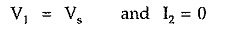Equation (i) can be written as,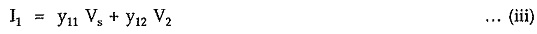Equation (ii) can be written as,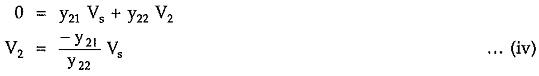Substituting value of V2 in equation (iii), we have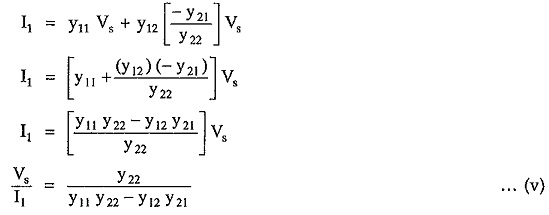Consider Fig. 6.6 (b).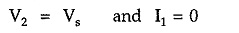Equation (i) can be written as,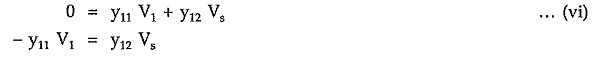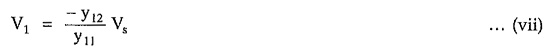Equation (ii) can be written as,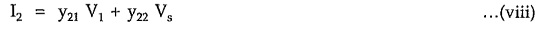Substituting value of V1 in equation (viii) from equation (vii), we have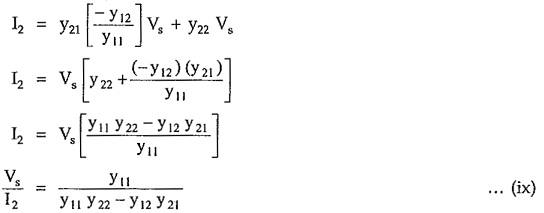For symmetrical networks, both the port impedances must be equal i.e.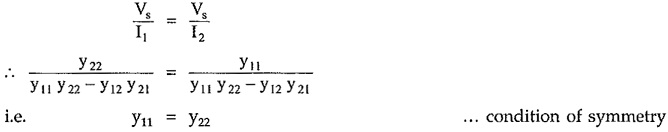#### Conditions of Symmetry for h-Parameters:

The basic equations of h-parameters are as follows,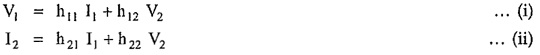Consider Fig. 6.6 (a),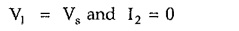Equation (i) can be written as,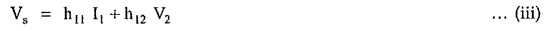Equation (ii) can be written as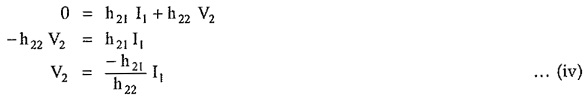Substituting value of V2 in equation (iii), we have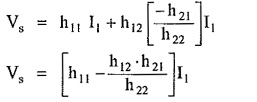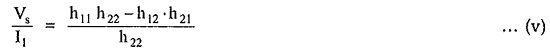Consider Fig. 6.6 (b),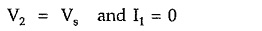Equation (i) can be written as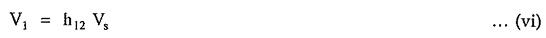Equation (ii) can be written as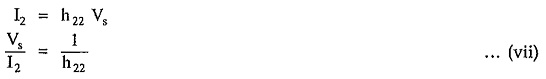For symmetrical networks, both the port impedances must be equal i.e.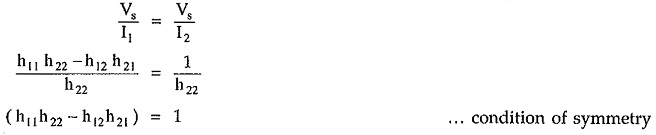#### Condition of Symmetry for Transmission Parameters:

The basic equations for the transmission parameters are as following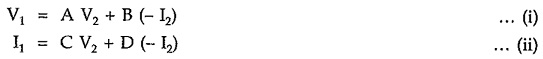Consider Fig. 6.6 (a),Equation (i) can be written as,Equation (ii) can be written as,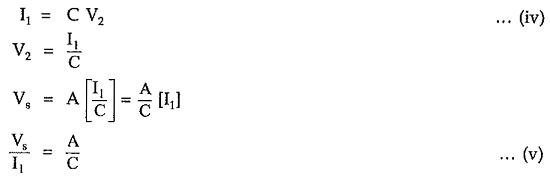Consider Fig. 6.6 (b),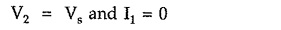Equation (i) can be written as,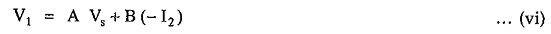Equation (ii) can be written as,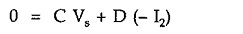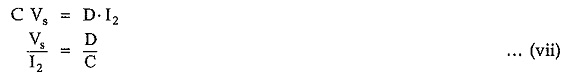For the symmetrical network, both the port impedances must be equal.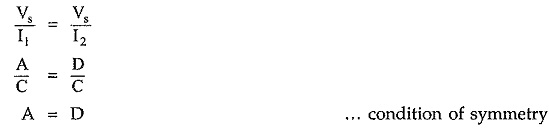Updated: November 5, 2019 — 10:32 pm